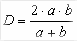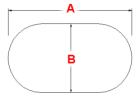Equivalent diameter for rectangular duct or oblong duct. Hydraulic diameter according to the Chesy's formula.
French site
| Home | Thematic | Tables | Programs | Library | Download | Links | Contact | Forum |--

Equivalent diameter
 Thematic Quotation Margin Hydraulic Linear pressure loss Local pressure loss Compressible fluids No circular duct Thermal Thermal fluid flow Pipe sizing steam Control valves Orifice plate Pumps Ventilators Gas Fuel gasHydraulic diameter

The formulas established for the calculation of pressure loss are established for ducts with circular section. It is the form best adapted, because it gives the greatest section for a given perimeter.

In practice such as for example for reasons of obstruction or esthetics, one meets other forms of networks, used in particular to convey air treated in the installations of distribution of air-conditioning.

One has for that resort to the concept of hydraulic diameter corresponding to the equivalent diameter or are really used in the case of a circular conduit.

Chesy established about 1820 a theoretical relation for ducts of an unspecified form:with:

• D = Diameter hydraulic
• S = Section of the duct
• P = Perimeter of the duct
 Rectangular ducts

On the basis of the Chesy's formula, the hydraulic diameter for a rectangular duct is calculated by means of the following expression:With:

• a = Width of the duct
• b = Height of the duct

If in turbulent flow the mean velocity is practically homogeneous in any point of the section of a conduit, in laminar flow that is much less.

It is recommended to show much circumspection when one plans to resort to the concept of diameter hydraulic in the case of a laminar flow.

For this reason, it is useful to use other formulas of computation based on the concept of equivalent diameter.

In the case of use of conduit rectangular, the equivalent diameter can be obtained for example by the expression of the ASHRAE:Oblong ducts

In the case of use of oblong ducts, the equivalent diameter is obtained by the expression of the ASHRAE:With:

• a = Width of the duct (in particular the large diameter for an oblong duct)
• b = Height of the duct (in particular the small diameter for an oblong duct)Last update: